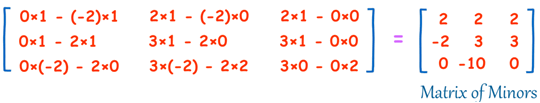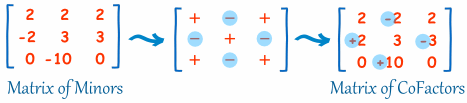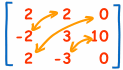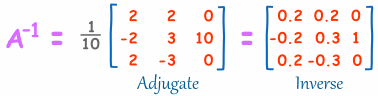C program to find Inverse of Matrix

C program to find Inverse of Matrix

76491
36
SHARE

C program to find Inverse of Matrix : For a square matrix A, the inverse matrix is written A-1. When A is multiplied by A-1 the result is the identity I. Non-square matrices do not have inverses. There are several different methods for calculating inverse of Matrix like Augmented Matrix Method, Adjoint Method and few shortcut methods. Today i have written a  to find Inverse of Square or say NxN Matrix using Adjoint Method.

Note: Not all square matrices have inverses. A square matrix which has an inverse is called invertible or non-singular, and a square matrix without an inverse is called non-invertible or singular.

Algorithm to calculate Inverse of Matrix using Adjoint Method :

1) calculating the Matrix of Minors

2) then turn that into the Matrix of Cofactors

4) multiply that by 1/Determinant.

But it is best explained by working through an example!

Example: find the Inverse of A:It needs 4 steps. It is all simple arithmetic but there is a lot of it, so try not to make a mistake!

Step 1: Matrix of Minors

The first step is to create a “Matrix of Minors”:

For each element of the matrix:

• ignore the values on the current row and column
• calculate the determinant of the remaining values

Put those determinants into a matrix (the “Matrix of Minors”)

Determinants

For a 2×2 matrix (2 rows and 2 columns) the determinant is easy: ad-bc

 Think of a cross: Blue means positive (+ad), Red means negative (-bc)The Calculations

Here are the first two, and last two, calculations of the “Matrix of Minors” (notice how I ignore the values in the current row and columns, and calculate the determinant using the remaining values):And here is the calculation for the whole matrix:Step 2: Matrix of Cofactors

This is easy! Just apply a “checkerboard” of minuses to the “Matrix of Minors”. In other words, you need to change the sign of alternate cells, like this:Now “Transpose” all elements of the previous matrix… in other words swap their positions over the diagonal (the diagonal stays the same):Step 4: Multiply by 1/Determinant

Now find the determinant of the original matrix. This isn’t too hard, because we already calculated the determinants of the smaller parts when we did “Matrix of Minors”.So: multiply the top row elements by their matching “minor” determinants:

Determinant = 3×2 – 0×2 + 2×2 = 10

And now multiply the Adjugate by 1/Determinant:And we are done!

Now let us see how to implement above explained method using C program which takes order of Square Matrix as input and then Matrix and displays Determinant and Inverse Matrix as output.

C Program to find Inverse of Matrix :

```#include<stdio.h>
#include<math.h>
#include<conio.h>
float determinant(float[][],float);
void cofactor(float[][],float);
void transpose(float[][],float[][],float);
int main()
{
float a,k,d;
int i,j;
printf("-------------------------------------------------------------\n");
printf("----------------made by C code champ ------------------------\n");
printf("-------------------------------------------------------------\n");
printf("\n  C Program to find inverse of Matrix\n\n");
printf("Enter the order of the Matrix : ");
scanf("%f",&k);
printf("Enter the elements of %.0fX%.0f Matrix : \n",k,k);
for (i=0;i<k;i++)
{
for (j=0;j<k;j++)
{
scanf("%f",&a[i][j]);
}
}
d=determinant(a,k);
printf("Determinant of the Matrix = %f",d);
if (d==0)
printf("\nInverse of Entered Matrix is not possible\n");
else
cofactor(a,k);
printf("\n\n**** Thanks for using the program!!! ****");
getch();
}

/*For calculating Determinant of the Matrix */
float determinant(float a,float k)
{
float s=1,det=0,b;
int i,j,m,n,c;
if (k==1)
{
return (a);
}
else
{
det=0;
for (c=0;c<k;c++)
{
m=0;
n=0;
for (i=0;i<k;i++)
{
for (j=0;j<k;j++)
{
b[i][j]=0;
if (i != 0 && j != c)
{
b[m][n]=a[i][j];
if (n<(k-2))
n++;
else
{
n=0;
m++;
}
}
}
}
det=det + s * (a[c] * determinant(b,k-1));
s=-1 * s;
}
}

return (det);
}

void cofactor(float num,float f)
{
float b,fac;
int p,q,m,n,i,j;
for (q=0;q<f;q++)
{
for (p=0;p<f;p++)
{
m=0;
n=0;
for (i=0;i<f;i++)
{
for (j=0;j<f;j++)
{
if (i != q && j != p)
{
b[m][n]=num[i][j];
if (n<(f-2))
n++;
else
{
n=0;
m++;
}
}
}
}
fac[q][p]=pow(-1,q + p) * determinant(b,f-1);
}
}
transpose(num,fac,f);
}
/*Finding transpose of matrix*/
void transpose(float num,float fac,float r)
{
int i,j;
float b,inverse,d;

for (i=0;i<r;i++)
{
for (j=0;j<r;j++)
{
b[i][j]=fac[j][i];
}
}
d=determinant(num,r);
for (i=0;i<r;i++)
{
for (j=0;j<r;j++)
{
inverse[i][j]=b[i][j] / d;
}
}
printf("\n\n\nThe inverse of matrix is : \n");

for (i=0;i<r;i++)
{
for (j=0;j<r;j++)
{
printf("\t%f",inverse[i][j]);
}
printf("\n");
}
}```

We hope you all have enjoyed the article on C program to find Inverse of NxN Matrix. If you have any issues ask me in form of comments.

References:

2. Mathisfun(Example)

1. I’ve a WordPress blog with Arras theme. This site strangely shows different on different computers. On some computers, I see all 3 columsn, on other PC, I see only 1. On other PCs, some wiered things. Please somebody help me..

2. Wow, wonderful blog layout! How long have you been blogging for? you make blogging look easy. The overall look of your website is fantastic, let alone the content!. Thanks For Your article about C Program to find inverse of matrix | Inverse of NxN Matrix .

3. sir i like the code since its nt too long nd works… but i will feel more comfortable if u add comments to make understand wht actually is happening in each n every line….

regards

4. The code is very difficult and solving of determinant of matrix can by written with recursion techniques (except other parts of code). Its looks like beginners programming trying.

5. this program 100% correct .
please fill up blank [] with 25 .
as like ……………….

#include
#include
#include
float determinant(float,float);
void cofactor(float,float);
void transpose(float,float,float);
int main()
{………………………………………………………………………………..

Tnx bro ,
solve this problem . I am new in c . A lot of good wish for U .

6. The programme in c about inverse of nxn matrix is not compiled when tested what could be the reason ?
bmsrao

7. Wow, amazing blog layout! How long have you been blogging for? you made blogging look easy. The overall look of your site is excellent, let alone the content!. Thanks For Your article about C Program to find inverse of matrix | Inverse of NxN Matrix .

8. first thank you for code , but when I try the code
it gives me an error like this
1>c:\users\ganem\documents\visual studio 2008\projects\fortest\fortest\fortest.cpp(83) : error C2371: ‘transpose’ : redefinition; different basic types

what does this error mean and i could solve this error

9. Well i have been trying to used to calculate determinant using this function by passing 2dimensional matrix of size 100 as an input,but the output i am not able to get instead displaying killed.
function determinant
if (i != 0 && j != c)
{
b[m][n]=a[i][j]; // this line is making as segmentation fault error
if (n<(k-2))
n++;
}

10. hi..
program works fine.
but matrix size is over 10, processing time is exponentially increase.
The matrix size is 25 How long does it take your time?
so..How I can be computed quickly?
thank you..

11. please good people can some1 help me with the c program to find the inverse of a 4*4 complex matrix. Thank you in advance

12. #include
#include
#include
float determinant(float[][],float);
void cofactor(float[][],float);
void transpose(float[][],float[][],float);
int main()
{
float a,k,d;
int i,j;
printf(“————————————————————-\n”);
printf(“—————-made by C code champ ————————\n”);
printf(“————————————————————-\n”);
printf(“\n C Program to find inverse of Matrix\n\n”);
printf(“Enter the order of the Matrix : “);
scanf(“%f”,&k);
printf(“Enter the elements of %.0fX%.0f Matrix : \n”,k,k);
for (i=0;i<k;i++)
{
for (j=0;j<k;j++)
{
scanf("%f",&a[i][j]);
}
}
d=determinant(a,k);
printf("Determinant of the Matrix = %f",d);
if (d==0)
printf("\nInverse of Entered Matrix is not possible\n");
else
cofactor(a,k);
printf("\n\n**** Thanks for using the program!!! ****");
getch();
}

/*For calculating Determinant of the Matrix */
float determinant(float a,float k)
{
float s=1,det=0,b;
int i,j,m,n,c;
if (k==1)
{
return (a);
}
else
{
det=0;
for (c=0;c<k;c++)
{
m=0;
n=0;
for (i=0;i<k;i++)
{
for (j=0;j<k;j++)
{
b[i][j]=0;
if (i != 0 && j != c)
{
b[m][n]=a[i][j];
if (n<(k-2))
n++;
else
{
n=0;
m++;
}
}
}
}
det=det + s * (a[c] * determinant(b,k-1));
s=-1 * s;
}
}

return (det);
}

void cofactor(float num,float f)
{
float b,fac;
int p,q,m,n,i,j;
for (q=0;q<f;q++)
{
for (p=0;p<f;p++)
{
m=0;
n=0;
for (i=0;i<f;i++)
{
for (j=0;j<f;j++)
{
if (i != q && j != p)
{
b[m][n]=num[i][j];
if (n<(f-2))
n++;
else
{
n=0;
m++;
}
}
}
}
fac[q][p]=pow(-1,q + p) * determinant(b,f-1);
}
}
transpose(num,fac,f);
}
/*Finding transpose of matrix*/
void transpose(float num,float fac,float r)
{
int i,j;
float b,inverse,d;

for (i=0;i<r;i++)
{
for (j=0;j<r;j++)
{
b[i][j]=fac[j][i];
}
}
d=determinant(num,r);
for (i=0;i<r;i++)
{
for (j=0;j<r;j++)
{
inverse[i][j]=b[i][j] / d;
}
}
printf("\n\n\nThe inverse of matrix is : \n");

for (i=0;i<r;i++)
{
for (j=0;j<r;j++)
{
printf("\t%f",inverse[i][j]);
}
printf("\n");
}
}

Read original Article: C Program to find inverse of matrix | Inverse of NxN Matrix

13. please can you help me to write a computer program that will accept an arbitrary n; the matrix A and right hand side b to solve the problem Ax = b by calculating the inverse of A and computing the solution of the system?

14. M =

852.1288 731.0261 611.8749 496.9069
731.0261 632.0341 532.6511 435.3030
611.8749 532.6511 453.2353 373.5039
496.9069 435.3030 373.5039 311.5798
I tested your program with matrix M. but the result is difference with result when I use command “inv(M)” in matlab. Please check your program and compare results to matlab. help me resolve this problem
– The result in matlab:
IM =

0.3960 -0.7607 0.3311 0.0342
-0.7607 1.8553 -1.4127 0.3146
0.3311 -1.4127 1.9886 -0.9383
0.0342 0.3146 -0.9383 0.6340

15. This code is not showing up the correct result for 2X2 matrix.
3 3.5
3.2 3.6
Help me with this test-case.